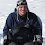# Learning Clojure

## Sunday, September 5, 2010

### Learn and Use the Standard Library

```;; What a lot of ways there are to write frequencies!

(defn freq-merge [sq]
(apply merge-with + (map (fn [c] {c 1}) sq)))

(defn freq-reduce-assoc [sq]
(reduce #(assoc %1 %2 (inc (get %1 %2 0))) {} sq))

(defn freq-reduce-update [sq]
(reduce #(update-in %1 [%2] (fnil inc 0)) {} sq))

(defn freq-loop [sq]
(loop [acc {} sq sq]
(if-let [sq (seq sq)]
(recur (assoc acc (first sq) (inc (get acc (first sq) 0))) (rest sq))
acc)))

;; Here's the source definition
(defn frequencies
"Returns a map from distinct items in coll to the number of times
they appear."
[coll]
(persistent!
(reduce (fn [counts x]
(assoc! counts x (inc (get counts x 0))))
(transient {}) coll)))

(def sq "abcdaabccc")
(time (frequencies sq))         "Elapsed time: 0.13 msecs"
(time (freq-loop sq))           "Elapsed time: 0.12 msecs"
(time (freq-reduce-assoc sq))   "Elapsed time: 0.13 msecs"
(time (freq-merge sq))          "Elapsed time: 0.20 msecs"
(time (freq-reduce-update sq))  "Elapsed time: 0.27 msecs"

(def sq (apply str (repeat 1000 "abcdaabccc")))
(time (frequencies sq))         "Elapsed time: 8  msecs"
(time (freq-loop sq))           "Elapsed time: 13 msecs"
(time (freq-reduce-assoc sq))   "Elapsed time: 13 msecs"
(time (freq-merge sq))          "Elapsed time: 42 msecs"
(time (freq-reduce-update sq))  "Elapsed time: 45 msecs"

(def sq (apply str (repeat 100000 "abcdaabccc")))
(time (frequencies sq))         "Elapsed time: 900  msecs"
(time (freq-loop sq))           "Elapsed time: 1500 msecs"
(time (freq-reduce-assoc sq))   "Elapsed time: 1500 msecs"
(time (freq-merge sq))          "Elapsed time: 5000 msecs"
(time (freq-reduce-update sq))  "Elapsed time: 5400 msecs"

;; Mumon's commentary: Learn and use the standard library

;; I don't know how many people read my ramblings, but if anyone notices places
;; where I could have used a library function, but have done it the hard way,
;; I'd consider it a great favour if you'd let me know.```

#### 1 comment:

1.great point. i had written a crazy (for) to piece together a string only to later find out that i had forgotten about str-join.